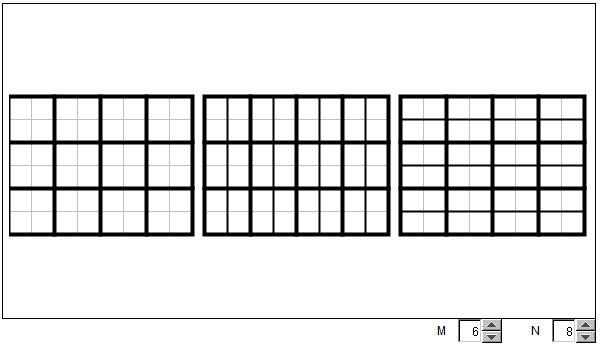# GCD(M, N) × LCM(M, N) = M × N

The applet below attempts to demonstrate an engaging property of GCD (the Greatest Common Divisor) and LCM (the Least Common Multiple) of two number, to boot,

 (1) GCD(M, N) × LCM(M, N) = M × N,

where M and N are two natural numbers.

Given two (natural) numbers M and N, we may form a grid M×N rectangle. Call it R. G = GCD(M, N) can be defined as the side length of the largest square that tessellates R. Indeed, GCD(M, N) is a factor of both M and N and is the largest number with this property.

In the applet, the leftmost M×N rectangle represents R tessellated by G×G squares. The number of such squares is

 M×NG×G
=
 MG
×
 NG

Call it S = (M/G)×(N/G). Obviously, S×G = M×(N/G) = (M/G)×N, so that S×G is divisible by both M and N. Call it L = S×G.

In the applet, there are two additional rectangles of the same size as R. These suggest the two ways in which L can be obtained: (M/G)×N and M×(N/G). In one, the G×G squares are split vertically into G×1 rectangles. In the other, they are split horizontally into 1×G rectangles. The total number of small rectangles in both cases is L.

### If you are reading this, your browser is not set to run Java applets. Try IE11 or Safari and declare the site https:///www.cut-the-knot.org as trusted in the Java setup.What if applet does not run?

I claim that L = LCM(M, N). For one, by the definition, L is divisible by both N and M. Necessarily, this is the least number with this property. Indeed, if it were otherwise, there would be rectangles K×1, bigger than G×1 that still tessellate R. But this would imply that, on one hand, K > G, and, on the other, that K divides both M and N, such that K ≤ G = GCD(M, N). Which is a contradiction. (This argument may benefit from extra finesse but is essentially correct.)

The relation GCD(M, N) × LCM(M, N) = M × N, has its root in the obvious identity

 (2) A + B = min(A, B) + max(A, B),

because, if the numbers are not equal, then one of them is smaller and one is larger than the other. (If the number are equal, the (2) holds vacuously.)

We shall use the prime number decomposition of N and M:

 (3) M = paqb· ... · rc, N = pαqβ· ... · rγ.

For example, for M = 12 and N = 10,

M = 223150,
N = 213051.

We see that it is always possible to write both M and N as the products of the same prime factors, albeit with different exponents. Some of the exponents will need to be zero.

Now, both GCD(M, N) and LCM(M, N) are products of the same prime factors where the exponents in G are the least of the corresponding two, whilst in L they are the largest:

GCD(M, N) = p min(a, α) q min(b, β)· ... · r min(c, γ),
LCM(M, N) = p max(a, α) q max(b, β)· ... · r max(c, γ).

Now, (2) thus insures that (1) holds. To continue the example:

GCD(12, 10) = 213050 = 2,
LCM(12, 10) = 223151 = 60.

Naturally, 12×10 = 2×60.• Factoring with the Factor Tree
• GCD and LCM via Factor Tree
• GCD and LCM by Plain Factorization
• Common Multiples and the Least Common Multiple
• GCD(M, N) x LCM(M, N) = M x N
• Divisibility Criteria
• Euclid's Algorithm
• Algorithm for Computing the LCM
• Factors And Multiples
• Two Properties of Greatest Common Divisor
• Properties of GCD and LCM
• A Line in a Square Grid
• Number of Factors of an Integer
• Extension of Euclid's Game
•